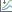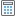# Parameter optimization

Disponible con una licencia de Geostatistical Analyst.

## Parameter optimization

Geostatistical Analyst offers the choice to optimize, via the Optimize button, the output for a range of interpolation models. In all these models, the optimization is based on minimizing the mean square error. The optimization process for each model is focused on its most important parameter(s).

The power value of IDW is the only parameter for this interpolation model used in the optimization.

The Kernel Parameter value is the only varying optimization parameter used with the Radial Basis Functions.

The Elevation Inflation Factor in Empirical Bayesian Kriging 3D can be optimized. The optimal value depends on many other parameters, so it is recommended to choose all other parameters before optimizing the elevation inflation factor.

Optimization is also used in kriging to assist in the fitting of the Semivariogram/covariance/cross-covariance model and is primarily focused on the range parameter. Semivariogram/covariance model optimization is performed assuming that one semivariogram model is used, that it is isotropic, and that the searching neighborhood is the default, with four sectors. The Shifts parameter used in cokriging can be included in the optimization process.

In LPI optimization, if the Advanced mode flag is set to False, the spatial condition number is not used in the optimization. The Goodness of Fit value can be used to determine an LPI model that produces optimal values.

It is also possible to optimize particular parameters for some of the interpolation models. In the case of LPI, the Bandwidth parameter and the Spatial Condition Number Threshold parameter can be optimized.

There are various semivariogram parameters in kriging that can be individually optimized by using the Automatic buttonin the Geostatistical Wizard. The mean square error for a series of values is calculated, and the parameter with the smallest mean square error value is selected.

##### En este tema
1. Parameter optimization